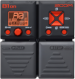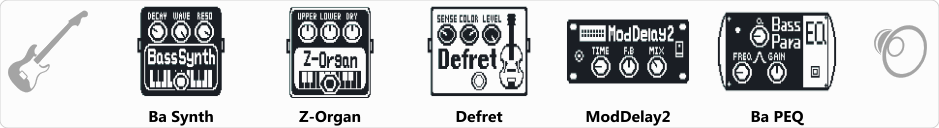# KL metheny

Discussion in 'Zoom B1on/B1Xon' started by Kovács László, Oct 15, 2018.

1. KL methenyDevice: Zoom B1on
Firmware: 1.21

Name on device: Matheny04
Optimized for: Phones/Speaker

Effects chain:For guitar synth. I like it.

Effect: "Ba Synth" (Modulation / Sfx), active - "yes"
"Decay" = 100
"Wave" = PWM
"Reso" = 2
"Synth" = 100
"Dry" = 0
"Level" = 135

Effect: "Z-Organ" (Modulation / Sfx), active - "yes"
"Upper" = 0
"Lower" = 0
"Dry" = 80
"HPF" = 3
"LPF" = 5
"Level" = 100

Effect: "Defret" (Modulation / Sfx), active - "yes"
"Sense" = 22
"Color" = 1
"Level" = 140
"Tone" = 41

Effect: "ModDelay2" (Delay / Reverb), active - "yes"
"Time" = 445
"F.B" = 27
"Mix" = 25
"Rate" = 2
"Level" = 100
"Depth" = 50
"Tail" = On

Effect: "Ba PEQ" (Dynamics / Filter), active - "yes"
"Freq1" = 4.0kHz
"Q1" = 1
"Gain1" = 20
"Freq2" = 2.5kHz
"Q2" = 1
"Gain2" = 19
"Level" = 57
Patch Volume: 50

Note: This is a patch file, you will need to download and install the ToneLib software to use the patch.

#### Attached Files:

• ###### KL_metheny.b1on
File size:
481 bytes
Views:
339
JamesBonze and DJ Bebop like this.
2. Thanks !